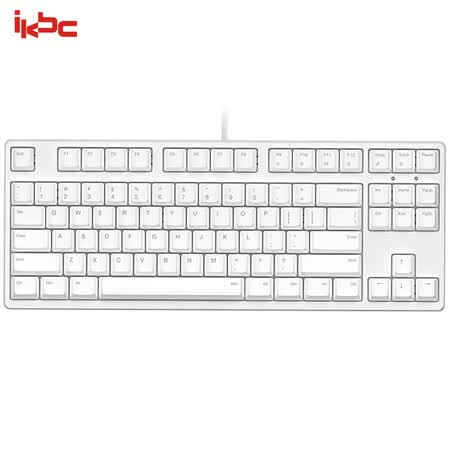# [译文]Java 和 Scala 的类型系统是不健全的

Java和Scala的类型系统使用了参数化多态（parametric polymorphism），因此允许带参数的函数根据参数来实现类型转换。作者同时指出因为JVM没有引入参数化多态，JVM不受此影响。最后，作者揭示了这样的问题在程序语言的设计上有着更大的影响。

2004年，Java 5 引入了泛型（generics）——参数化多态；同年Scala发布，发布了path-dependent type的特性。在发布之初，二者的类型系统都是不健全的。尽管诸多PL研究者对于Java的类型系统进行研究，并将其形式化，作者还是发现了Java类型系统不健全的地方。

``````class Unsound {
static class Constrain<A, B extends A> {}
static class Bind<A> {
<B extends A>
A upcast(Constrain<A,B> constrain, B b) {
return b;
}
}
static <T,U> U coerce(T t) {
Constrain<U,? super T> constrain = null;
Bind<U> bind = new Bind<U>();
return bind.upcast(constrain, t);
}
public static void main(String[] args) {
String zero = Unsound.<Integer,String>coerce(0);
}
}
``````

Java 8运行结果（`ClassCastException`）：

``````\$ javac -version
javac 1.8.0_151
\$ javac Unsound.java
\$ java Unsound
Exception in thread "main" java.lang.ClassCastException: java.lang.Integer cannot be cast to java.lang.String
at Unsound.main(Unsound.java:15)
``````

Java 9运行结果（无法编译）：

``````\$ javac -version
javac 9.0.1
\$ javac Unsound.java
Unsound.java:12: error: method upcast in class Bind<A> cannot be applied to given types;
return bind.upcast(constrain, t);
^
required: Constrain<U,B>,B
found: Constrain<U,CAP#1>,T
reason: inference variable B has incompatible bounds
upper bounds: U
lower bounds: T
where U,T,B,A are type-variables:
U extends Object declared in method <T,U>coerce(T)
T extends Object declared in method <T,U>coerce(T)
B extends U declared in method <B>upcast(Constrain<A,B>,B)
A extends Object declared in class Bind
where CAP#1 is a fresh type-variable:
CAP#1 extends U super: T from capture of ? super T
1 error
``````

``````class A {}
class B {}
class Unsound {
<V, W> V magic(W w) {
// TODO
}
public static void main(String[] args) {
Unsound u = new Unsound();
A surprise = u.<A, B>magic(new B()); // 把B转换到A
}
}
``````

`magic`方法里，我们需要一个`V`的实例，但我们只有一个`W`的实例，显然我们没有办法完成给定的任务。但是我们可以使用通配符给类型进行限制，然后进行安全的向上类型转换（upcast）。

``````class Constrain<X, Y extends X> {}
class Bind<Z> {
<U extends Z> Z
upcast(Constrain<Z, U> constrain, U u) { return u; }
}
``````

``````<V, W> V magic(W w) {
Constrain<???> constrain = ???; //TODO
Bind<V> bind = new Bind<V>();
return bind.upcast(constrain, w);
}
``````

• `Constrain<T1, T2>` 是一个合法的类型
• `bind.upcast`可以通过类型检查
• `val`满足`Constrain<T1, T2>`的类型限制

``````Constrain<V, ? super W> constrain = null;
``````

1. `U` = `X` – 通过归一`Constrain<V, U>``Constrain<V, X>`
2. `W``U`的子类 – 通过第二个参数的类型，`w`必须可以被转换到`U`
3. `U``V`的子类 – 函数定义中`U extends Z``Z`被显式指定为`V`

（1）荒谬的类型

`String` <: `Top` <: `V` <: `Bottom` <: `Integer`

（2）`null` —— the billion dollar mistake

（3）没有预见的特性交互